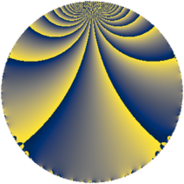# Properties

 Label 70.2.kLevel $70$ Weight $2$ Character orbit 70.k Rep. character $\chi_{70}(3,\cdot)$ Character field $\Q(\zeta_{12})$ Dimension $16$ Newform subspaces $1$ Sturm bound $24$ Trace bound $0$

# Related objects

## Defining parameters

 Level: $$N$$ $$=$$ $$70 = 2 \cdot 5 \cdot 7$$ Weight: $$k$$ $$=$$ $$2$$ Character orbit: $$[\chi]$$ $$=$$ 70.k (of order $$12$$ and degree $$4$$) Character conductor: $$\operatorname{cond}(\chi)$$ $$=$$ $$35$$ Character field: $$\Q(\zeta_{12})$$ Newform subspaces: $$1$$ Sturm bound: $$24$$ Trace bound: $$0$$

## Dimensions

The following table gives the dimensions of various subspaces of $$M_{2}(70, [\chi])$$.

Total New Old
Modular forms 64 16 48
Cusp forms 32 16 16
Eisenstein series 32 0 32

## Trace form

 $$16q - 12q^{5} + 8q^{7} + O(q^{10})$$ $$16q - 12q^{5} + 8q^{7} - 12q^{10} - 12q^{11} + 16q^{15} + 8q^{16} - 36q^{17} - 8q^{18} - 28q^{21} - 8q^{22} - 4q^{23} + 12q^{25} + 12q^{26} + 4q^{28} + 20q^{30} + 24q^{31} + 48q^{33} + 8q^{35} - 8q^{36} + 4q^{37} + 24q^{38} + 36q^{42} - 8q^{43} - 12q^{45} - 8q^{46} + 12q^{47} - 32q^{50} - 16q^{51} - 28q^{53} - 4q^{56} + 8q^{57} - 32q^{58} + 8q^{60} - 12q^{61} - 36q^{63} - 8q^{65} + 32q^{67} - 36q^{68} - 12q^{70} + 16q^{71} - 8q^{72} - 12q^{73} - 48q^{75} + 16q^{77} + 16q^{78} - 12q^{80} - 48q^{82} + 24q^{85} + 12q^{86} - 24q^{87} - 4q^{88} - 16q^{91} + 8q^{92} + 28q^{93} + 20q^{95} + 12q^{96} + 40q^{98} + O(q^{100})$$

## Decomposition of $$S_{2}^{\mathrm{new}}(70, [\chi])$$ into newform subspaces

Label Dim. $$A$$ Field CM Traces $q$-expansion
$$a_2$$ $$a_3$$ $$a_5$$ $$a_7$$
70.2.k.a $$16$$ $$0.559$$ $$\mathbb{Q}[x]/(x^{16} + \cdots)$$ None $$0$$ $$0$$ $$-12$$ $$8$$ $$q+(-\beta _{7}-\beta _{15})q^{2}+(-\beta _{4}+\beta _{13})q^{3}+\cdots$$

## Decomposition of $$S_{2}^{\mathrm{old}}(70, [\chi])$$ into lower level spaces

$$S_{2}^{\mathrm{old}}(70, [\chi]) \cong$$ $$S_{2}^{\mathrm{new}}(35, [\chi])$$$$^{\oplus 2}$$